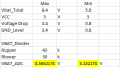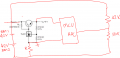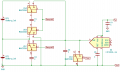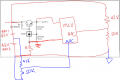# ADC Voltage Measurement for shifted DC GND using microcontroller - Help Needed

#### mishra87

Joined Jan 17, 2016
958
Hi Friend,

I have two series battery with top of charge 8.4V(4.2V each cell). Battery lower cut off voltage is 5.8V.
So input supply is 5.8V to 8.4V and i have a few mA load for 5V supply as per below.
My rest of input supply voltage from battery will be drop by series resistor R or some other components.

I made some calculation here for ADC measurement but i am not sure if i am correct here. As per my assumption if 5V is output then rest of battery voltage will be dropped by resistor so GND level for microcontroler is shifted somewhere as per below.

My objective here to measure both battery cell voltage individually but i am not sure how this will be done.

How Much and how microcontroller will read ADC value. Divider voltage is 4.5V for 8.4V battery and 3.15 for 5.8V battery.
Could anyone explain here ?

This calculation is for total battery voltage. Is it possible to measure individual battery voltage for both cells ?#### geekoftheweek

Joined Oct 6, 2013
814
How may ADCs do you have? Connect another between the batteries. Since you already have the total voltage the second ADC could give the voltage of the bottom battery and subtract it from the total for the top.

#### mishra87

Joined Jan 17, 2016
958
Yes, I know this concept !

How ADC measurement will be done in case of shifted GND level.
May me you have look the schematic about battery GND and microcontroller GND

Thanks !

#### schmitt trigger

Joined Jul 12, 2010
496
Perhaps you should start by explaining to us why, in the first place, are you shifting the ground?

•ronsimpson

#### mishra87

Joined Jan 17, 2016
958
Perhaps you should start by explaining to us why, in the first place, are you shifting the ground?
Ideally there should not GND shifting.
I am not developing this circuit. This is already designed circuit and i am doing some modification on this circuit.

I wanted to measure voltage of both cell individually for protection but i do not understand measure concept of this circuit using ADC since GND is shifted.

Thanks !

#### crutschow

Joined Mar 14, 2008
29,752
How much is the ground shifted?
What is causing the ground shift?

#### mishra87

Joined Jan 17, 2016
958

#### crutschow

Joined Mar 14, 2008
29,752
Resistor R are in series.
Looks like we're in a game of 20 questions.

So what is the purpose of "R" and what is its value.
A random value for R doesn't tell us much.

#### mishra87

Joined Jan 17, 2016
958
Looks like we're in a game of 20 questions.

So what is the purpose of "R" and what is its value.
A random value for R doesn't tell us much.
Thanks,

I am not sure if i was able to explain correctly !
The value of R=130R

It can be understood . 5V will be from shunt regulator & rest is resistor drop !

Please let me know what all information you need. I do not know all information needed more.
Did you get change to look post 1 schematic.

Regards

#### Irving

Joined Jan 30, 2016
2,662
You can't shift the ground using R in your circuit because the current through R is indeterminate, it will depend on current drawn by MCU and absolute battery volts.

Using multiple stacked ADCs is one option but it has problems in terms of powering each ADC and level shifting the digital I2C side.

If you look at the internal circuits for battery monitors for EV where they have cell stacks to 100s of volts they use a technique called flying sample-hold or flying capacitor. It works by charging a capacitor from the cell in question and then transferring that charge down to a ground reference. The diagram below illustrates how its done...U1 is a quad SPDT analog switch. C1 charges to the voltage across BT1, C2 to that across BT2. A pulse on Clear ensures C3 is discharged. A pulse on Sample1 transfers the charge from C1 to C3, effectively shifting the value of BT1 to a ground reference. The ADC takes a reading for BT1. Clear is pulsed to zero C3 then Sample2 is pulsed to transfer the charge on C2 to C3, and the ADC takes another reading for BT2.

I've used this approach to monitor an 8 cell stack with analog switches rated at 40v. The MAX333A is good to 30v. Layout is important to avoid unnecessary leakage and C1 - C3 need to be good quality caps. From memory, I used 1nF which gives a 99.9% time constant of 110nS with the resistance of the switches and a 10uS conversion time, though the sample rate was much lower at 100 sps

•Deleted member 115935

#### mishra87

Joined Jan 17, 2016
958
You can't shift the ground using R in your circuit because the current through R is indeterminate, it will depend on current drawn by MCU and absolute battery volts.

Using multiple stacked ADCs is one option but it has problems in terms of powering each ADC and level shifting the digital I2C side.

If you look at the internal circuits for battery monitors for EV where they have cell stacks to 100s of volts they use a technique called flying sample-hold or flying capacitor. It works by charging a capacitor from the cell in question and then transferring that charge down to a ground reference. The diagram below illustrates how its done...

View attachment 211380
U1 is a quad SPDT analog switch. C1 charges to the voltage across BT1, C2 to that across BT2. A pulse on Clear ensures C3 is discharged. A pulse on Sample1 transfers the charge from C1 to C3, effectively shifting the value of BT1 to a ground reference. The ADC takes a reading for BT1. Clear is pulsed to zero C3 then Sample2 is pulsed to transfer the charge on C2 to C3, and the ADC takes another reading for BT2.

I've used this approach to monitor an 8 cell stack with analog switches rated at 40v. The MAX333A is good to 30v. Layout is important to avoid unnecessary leakage and C1 - C3 need to be good quality caps. From memory, I used 1nF which gives a 99.9% time constant of 110nS with the resistance of the switches and a 10uS conversion time, though the sample rate was much lower at 100 sps
You at least understand what i was trying to ask.

In your circuit , battery GND and ADC GND are same but not in mine.

The circuit in post is already implemented and it is in production. There is no scope to increase BOM cost so i was trying to measure battery voltage using resistor divider.

Total voltage of two cell battery as per post 1 is already implemented !

So my objective was to understand :

1. How ADC voltage measurement is being done ! Since microntroller GND is at higher level than battery GND.
2. How can i measure both cell battery voltage individually. so my idea was measure 2nd cell voltage and calculate 1st cell voltage usinf this formula :
1st cell voltage = Total voltage (step1) - 2nd cell voltage (step2)

I wanna make sure step1 is working but i do not understand ADC measurement method.
Is is possible to measure 2nd cell voltage as GND is higher level of microcontroller.#### Irving

Joined Jan 30, 2016
2,662
the ADC input must not go -ve wrt mcu gnd else bad things might happen. But thats not your problem. I think that circuit is very marginal over battery volts, temperature, etc. Here's my thinking... based on educated guesses...

Vtop (Top of battery stack wrt MCU gnd) = 5v + Vce of bypass transistor, and must be >> Vo + Vbe = 5.7v ideally Vce > 1v, ie Vtop >6v.

Vbot (Bottom of battery stack) = Vtop - Vbat. Vbat will be 2 x 3.7v @ 100%SOC -> 2 x 2.9v @ terminal SOC. It won't be 8.4v except for a few moments immediately after charge, it will fall to 2 x 3.7v within a few tens of seconds as soon as you take any current.

Ir (current through R) = current through MCU + current though TL431 = Imcu + Iak

Imcu = ???
Ika = (Vtop - 5.7)/Ro - Ib = (Vtop - 5.7)/Ro - Imcu/hfe

hfe could be 100 - 300 or more
Imcu unknown, anything from 1mA to 100mA or more depending on what mcu drives and other peripherals
Ika must be >100uA and <100mA, would typically be chosen around 5 to 10 x Ib(max)

Lets say Imcu = 20mA & hfe =200 then Ib = 0.1mA and Ika = 0.5mA. Typically Ro is 470 - 1k which means Vtop = 6 - 6.3v and Vce = 1 to 1.3v so thats a good starting guess...

If R = 130 then:
@Vbat = 8.4v and Vce = 1v then V across R must be 8.4 - 5 - 1 = 2.4v therefore Ir = 18mA
@Vbat = 7.4v and Ir = 18mA, Vce = 7.4 -5 -0.018 * 130 = 0.06! out of regulation, theres not enough headroom for TL431 to regulate.

try Imcu = 10mA
@Vbat = 8.4v and Vce = 2v then Vr = 1.4, Ir = 11mA
@Vbat = 7.4 and Ir = 11mA, Vce = 7.4 - 5 - .011 * 130 = 1v
@Vbat = 6.4 and Ir = 11mA Vce = 0v out of regulation.

Basically with R = 130 your circuit is very marginal

#### crutschow

Joined Mar 14, 2008
29,752
I still see no good reason for the shifted ground but, if you must have that 130 ohm resistor in there, then you also need to measure the battery ground voltage as well.
So you need to measure the voltage at the top of BAT1, at the junction of the two batteries, and at the bottom of BAT2.
From that you can calculate the voltage of the two batteries.

Last edited:

#### crutschow

Joined Mar 14, 2008
29,752
Why not use a Howland Current pump? If you are only measuring positive voltages, then the Op-Amps can be SIngle rail.
Why would you use a current-pump for measuring a voltage?#### Irving

Joined Jan 30, 2016
2,662
He can't measure the bottom end of the stack as its below the mcu ground.

If the current in R was <20mA then replacing R with a precision 1v reference might help. Then the top of 'R', MCU ground, would be fixed at 1v above the bottom of the battery. So now the junction of the cells can be measured as between 3.2 and 1.9v and the lower cell is that measurement plus 1v. The top cell voltage can be inferred as the total voltage (via voltage divider) less bottom cell voltage.

Doesn't solve the voltage regulation issue, it will still go out of regulation at a battery voltage of 6 - 6.3v, well above the 5.8v required.

#### crutschow

Joined Mar 14, 2008
29,752
He can't measure the bottom end of the stack as its below the mcu ground.
He could convert that negative voltage to a positive voltage with an inverting opamp.

#### mishra87

Joined Jan 17, 2016
958
He can't measure the bottom end of the stack as its below the mcu ground.

If the current in R was <20mA then replacing R with a precision 1v reference might help. Then the top of 'R', MCU ground, would be fixed at 1v above the bottom of the battery. So now the junction of the cells can be measured as between 3.2 and 1.9v and the lower cell is that measurement plus 1v. The top cell voltage can be inferred as the total voltage (via voltage divider) less bottom cell voltage.

Doesn't solve the voltage regulation issue, it will still go out of regulation at a battery voltage of 6 - 6.3v, well above the 5.8v required.
Y
He can't measure the bottom end of the stack as its below the mcu ground.

If the current in R was <20mA then replacing R with a precision 1v reference might help. Then the top of 'R', MCU ground, would be fixed at 1v above the bottom of the battery. So now the junction of the cells can be measured as between 3.2 and 1.9v and the lower cell is that measurement plus 1v. The top cell voltage can be inferred as the total voltage (via voltage divider) less bottom cell voltage.

Doesn't solve the voltage regulation issue, it will still go out of regulation at a battery voltage of 6 - 6.3v, well above the 5.8v required.
He could convert that negative voltage to a positive voltage with an inverting opamp.
Thanks all for keep on addressing the issue. !

As i understand we can not use opamp for negative voltage measurement why because opamp negative supply will be GND level which will above the measured signal. So signal below this GND will be 0V for opamp.

This idea may be implemented :

2nd Cell voltage = Total cell voltage(1st cell Positive w.r.t 2nd cell negative) - 1st cell voltage (VBAT1 w.r.t. junction of 2nd cell)

Yes, it is tricky circuit to implement !

Thanks again !

#### mishra87

Joined Jan 17, 2016
958
the ADC input must not go -ve wrt mcu gnd else bad things might happen. But thats not your problem. I think that circuit is very marginal over battery volts, temperature, etc. Here's my thinking... based on educated guesses...

Vtop (Top of battery stack wrt MCU gnd) = 5v + Vce of bypass transistor, and must be >> Vo + Vbe = 5.7v ideally Vce > 1v, ie Vtop >6v.

Vbot (Bottom of battery stack) = Vtop - Vbat. Vbat will be 2 x 3.7v @ 100%SOC -> 2 x 2.9v @ terminal SOC. It won't be 8.4v except for a few moments immediately after charge, it will fall to 2 x 3.7v within a few tens of seconds as soon as you take any current.

Ir (current through R) = current through MCU + current though TL431 = Imcu + Iak

Imcu = ???
Ika = (Vtop - 5.7)/Ro - Ib = (Vtop - 5.7)/Ro - Imcu/hfe

hfe could be 100 - 300 or more
Imcu unknown, anything from 1mA to 100mA or more depending on what mcu drives and other peripherals
Ika must be >100uA and <100mA, would typically be chosen around 5 to 10 x Ib(max)

Lets say Imcu = 20mA & hfe =200 then Ib = 0.1mA and Ika = 0.5mA. Typically Ro is 470 - 1k which means Vtop = 6 - 6.3v and Vce = 1 to 1.3v so thats a good starting guess...

If R = 130 then:
@Vbat = 8.4v and Vce = 1v then V across R must be 8.4 - 5 - 1 = 2.4v therefore Ir = 18mA
@Vbat = 7.4v and Ir = 18mA, Vce = 7.4 -5 -0.018 * 130 = 0.06! out of regulation, theres not enough headroom for TL431 to regulate.

try Imcu = 10mA
@Vbat = 8.4v and Vce = 2v then Vr = 1.4, Ir = 11mA
@Vbat = 7.4 and Ir = 11mA, Vce = 7.4 - 5 - .011 * 130 = 1v
@Vbat = 6.4 and Ir = 11mA Vce = 0v out of regulation.

Basically with R = 130 your circuit is very marginal
It is not necessary to have microcontroller supply voltage 5V. it can be rduced to 4V as microcontoller(STM8S) is having good input supply range to get ride of regulation problem.

I still do not understand why do you consider Vce = 1-2V because in saturation transistor Vce = 0.3Vmax.
Yes Vtop > VDD+0.7V

Lets do math for Imcu = 10mA and other board current is 5mA (including TL431)
I_total = 15max (this is current consumption analysis of my board)

Vtop(total) = 8.4V lets say 100% SOC of battery and 5.8V at min SOC
VDD = 4V

When Vtop = 8.4V, VDD = 4V
VR = (8.4V-4.7V) = 3.7V (voltage drop across resistor)
So when Vtop = 8.4, GND_MCU = 3.7V and now MCU will not measure signal below 3.7V

When Vtop 5.8V, VDD =4V
VR = 1.8V so in this case MCU will not measure voltage below 1.8V

Still i am confused how to do this ?

#### geekoftheweek

Joined Oct 6, 2013
814
Yes, I know this concept !

How ADC measurement will be done in case of shifted GND level.
May me you have look the schematic about battery GND and microcontroller GND

Thanks !
I just realized I made a huge error in not seeing the resistor and apologize for my response. It is still though a basic math situation if you look at it without the shifted ground in mind. Take the total voltage and subtract the voltage between the two batteries for bat 1. Then subtract bat 1 volts from total to get bat 2 voltage. I think that is where your last thinking was heading. Good luck!!

Edit... I just realized how the shifted ground would come in to play. I smacked myself on the back of the head for you!!

Last edited: